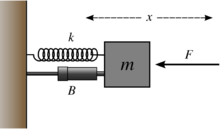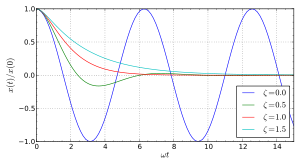# Damping

DampingDamped spring–mass system

In physics, damping is any effect that tends to reduce the amplitude of oscillations in an oscillatory system, particularly the harmonic oscillator.

In mechanics, friction is one such damping effect. For many purposes the frictional force Ff can be modeled as being proportional to the velocity v of the object:$F_{\mathrm f} = -c v \, ,$

where c is the viscous damping coefficient, given in units of newton seconds per meter (N s/m) or simply kilograms per second.

Generally, damped harmonic oscillators satisfy the second-order differential equation:$\frac{d^2x}{dt^2} + 2\zeta\omega_0\frac{dx}{dt} + \omega_0^2 x = 0,$

where ω0 is the undamped angular frequency of the oscillator and ζ is a constant called the damping ratio.

The value of the damping ratio ζ determines the behavior of the system. A damped harmonic oscillator can be:

• Overdamped (ζ > 1): The system returns (exponentially decays) to equilibrium without oscillating. Larger values of the damping ratio ζ return to equilibrium slower.
• Critically damped (ζ = 1): The system returns to equilibrium as quickly as possible without oscillating. This is often desired for the damping of systems such as doors.
• Underdamped (0 < ζ < 1): The system oscillates (at reduced frequency compared to the undamped case) with the amplitude gradually decreasing to zero.
• Undamped (ζ = 0): The system oscillates at its natural resonant frequency (ωo).

## Definition

In physics and engineering, damping may be mathematically modelled as a force synchronous with the velocity of the object but opposite in direction to it. If such force is also proportional to the velocity, as for a simple mechanical viscous damper (dashpot), the force F may be related to the velocity v by$F = -cv \, ,$

where c is the viscous damping coefficient, given in units of newton-seconds per meter.

This force is an approximation to the friction caused by drag.

This relationship is perfectly analogous to electrical resistance as described by Ohm's law.

## Example: mass–spring–damperA mass attached to a spring and damper. The damping coefficient, usually c, is represented by B in this case. The F in the diagram denotes an external force, which this example does not include.

An ideal mass–spring–damper system with mass m, spring constant k and viscous damper of damping coefficient c is subject to an oscillatory force$F_\mathrm{s} = - k x \,$

and a damping force$F_\mathrm{d} = - c v = - c \frac{dx}{dt} = - c \dot{x}.$

The values can be in any consistent system of units; for example, in SI units, m in kilograms, k in newtons per meter, and c in newton-seconds per meter or kilograms per second.

Treating the mass as a free body and applying Newton's second law, the total force Ftot on the body is$F_\mathrm{tot} = ma = m \frac{d^2x}{dt^2} = m \ddot{x}.$

where a is the acceleration of the mass and x is the displacement of the mass relative to a fixed point of reference.

Since Ftot = Fs + Fd,$m \ddot{x} = -kx + -c\dot{x}.$

This differential equation may be rearranged into$\ddot{x} + { c \over m} \dot{x} + {k \over m} x = 0.\,$

The following parameters are then defined:$\omega_0 = \sqrt{ k \over m }$$\zeta = { c \over 2 \sqrt{m k} }.$

The first parameter, ω0, is called the (undamped) natural frequency of the system . The second parameter, ζ, is called the damping ratio. The natural frequency represents an angular frequency, expressed in radians per second. The damping ratio is a dimensionless quantity.

The differential equation now becomes$\ddot{x} + 2 \zeta \omega_0 \dot{x} + \omega_0^2 x = 0.\,$

Continuing, we can solve the equation by assuming a solution x such that:$x = e^{\gamma t}\,$

where the parameter γ (gamma) is, in general, a complex number.

Substituting this assumed solution back into the differential equation gives$\gamma^2 + 2 \zeta \omega_0 \gamma + \omega_0^2 = 0 \, ,$

which is the characteristic equation.

Solving the characteristic equation will give two roots, γ+ and γ. The solution to the differential equation is thus$x(t) = Ae^{\gamma_+ t} + Be^{\gamma_- t} \, ,$

where A and B are determined by the initial conditions of the system:$A = x(0)+\frac{\gamma_+x(0)-\dot{x}(0)}{\gamma_--\gamma_+}$$B = -\frac{\gamma_+x(0)-\dot{x}(0)}{\gamma_--\gamma_+}.$

### System behaviorDependence of the system behavior on the value of the damping ratio ζ, for undamped (blue), under-damped (green), critically damped (red), and over-damped (cyan) cases, for zero-velocity initial condition.

The behavior of the system depends on the relative values of the two fundamental parameters, the natural frequency ω0 and the damping ratio ζ. In particular, the qualitative behavior of the system depends crucially on whether the quadratic equation for γ has one real solution, two real solutions, or two complex conjugate solutions.

#### Critical damping (ζ = 1)

When ζ = 1, there is a double root γ (defined above), which is real. The system is said to be critically damped. A critically damped system converges to zero as fast as possible without oscillating. An example of critical damping is the door closer seen on many hinged doors in public buildings. The recoil mechanisms in most guns are also critically damped so that they return to their original position, after the recoil due to firing, in the least possible time.

In this case, with only one root γ, there is in addition to the solution x(t) = eγt a solution x(t) = teγt:$x(t) = (A+Bt)\,e^{-\omega_0 t} \,$

where A and B are determined by the initial conditions of the system (usually the initial position and velocity of the mass):$A = x(0) \,$$B = \dot{x}(0)+\omega_0x(0) \,$

#### Over-damping (ζ > 1)

When ζ > 1, the system is over-damped and there are two different real roots. An over-damped door-closer will take longer to close than a critically damped door would.

The solution to the motion equation is:$x(t) = Ae^{\gamma_+ t} + Be^{\gamma_- t}$

where A and B are determined by the initial conditions of the system:$A = x(0)+\frac{\gamma_+x(0)-\dot{x}(0)}{\gamma_--\gamma_+}$$B = -\frac{\gamma_+x(0)-\dot{x}(0)}{\gamma_--\gamma_+}.$

#### Under-damping (0 ≤ ζ < 1)

Finally, when 0 ≤ ζ < 1, γ is complex, and the system is under-damped. In this situation, the system will oscillate at the natural damped frequency ωd, which is a function of the natural frequency and the damping ratio. To continue the analogy, an underdamped door closer would close quickly, but would hit the door frame with significant velocity, or would oscillate in the case of a swinging door.

In this case, the solution can be generally written as:$x (t) = e^{- \zeta \omega_0 t} (A \cos\,(\omega_\mathrm{d}\,t) + B \sin\,(\omega_\mathrm{d}\,t ))\,$

where$\omega_\mathrm{d} = \omega_0 \sqrt{1 - \zeta^2 }\,$

represents the damped frequency or ringing frequency of the system, and A and B are again determined by the initial conditions of the system:$A = x(0)\,$$B = \frac{1}{\omega_\mathrm{d}}(\zeta\omega_0x(0)+\dot{x}(0)).\,$

This "damped frequency" is not to be confused with the damped resonant frequency or peak frequency ωpeak. This is the frequency at which a moderately underdamped (ζ < 1/2) simple 2nd-order harmonic oscillator has its maximum gain (or peak transmissibility) when driven by a sinusoidal input. The frequency at which this peak occurs is given by:$\omega_{peak} = \omega_0\sqrt{1 - 2\zeta^2}.$

For an under-damped system, the value of ζ can be found by examining the logarithm of the ratio of succeeding amplitudes of a system. This is called the logarithmic decrement.

## Alternative models

Viscous damping models, although widely used, are not the only damping models. A wide range of models can be found in specialized literature, but one of them should be referred here: the so called "hysteretic damping model" or "structural damping model".

When a metal beam is vibrating, the internal damping can be better described by a force proportional to the displacement but in phase with the velocity. In such case, the differential equation that describes the free movement of a single-degree-of-freedom system becomes:$m \ddot{x} + h x i + k x = 0$

where h is the hysteretic damping coefficient and i denotes the imaginary unit; the presence of i is required to synchronize the damping force to the velocity (xi being in phase with the velocity). This equation is more often written as:$m \ddot{x} + k ( 1 + i \eta ) x = 0$

where η is the hysteretic damping ratio, that is, the fraction of energy lost in each cycle of the vibration.

Although requiring complex analysis to solve the equation, this model reproduces the real behaviour of many vibrating structures more closely than the viscous model.

A more general model that also requires complex analysis, the fractional model not only includes both the viscous and hysteretic models, but also allows for intermediate cases (useful for some polymers):$m \ddot{x} + A \frac{d^r x}{dt^r} i + k x = 0$

where r is any number, usually between 0 (for hysteretic) and 1 (for viscous), and A is a general damping (h for hysteretic and c for viscous) coefficient.

## See also

Wikimedia Foundation. 2010.

### Look at other dictionaries:

• Damping — Damping. См. Демпфирование. (Источник: «Металлы и сплавы. Справочник.» Под редакцией Ю.П. Солнцева; НПО Профессионал , НПО Мир и семья ; Санкт Петербург, 2003 г.) …   Словарь металлургических терминов

• Damping —   [engl.], Dämpfung …   Universal-Lexikon

• dàmping — (dèmping) m 〈N mn nzi, G dȁmpīngā〉 trg. prodaja robe ili usluge na tržištu po umjetno sniženim cijenama, radi eliminiranja konkurencije i osvajanja novog tržišta ✧ {{001f}}engl …   Veliki rječnik hrvatskoga jezika

• damping — dàmping (dèmping) m <N mn nzi, G dȁmpīngā> DEFINICIJA trg. prodaja robe ili usluge na tržištu po umjetno sniženim cijenama, radi eliminiranja konkurencije i osvajanja novog tržišta ETIMOLOGIJA engl. dumping: bacanje jeftine robe na tržište …   Hrvatski jezični portal

• damping — damp·ing (dămʹpĭng) n. The capacity built into a mechanical or electrical device to prevent excessive correction and the resulting instability or oscillatory conditions. * * * In physics, the restraint of vibratory motion, such as mechanical… …   Universalium

• damping — 1) (energy absorption) a shock absorber characteristic which is determined by the orifice pattern of the shock absorber. Dashpot (spike force), conventional (constant force), progressive (increasing force) and self compensating (various force)… …   Mechanics glossary

• Damping — El damping es una técnica musical a través de la cual se altera el sonido de un instrumento musical. Existen toda una serie de métodos de damping en función del tipo de instrumento Contenido 1 Guitarra 2 Piano 3 Véase también …   Wikipedia Español

• Damping — Damp Damp, v. i. [imp. & p. p. {Damped}; p. pr. & vb. n. {Damping}.] [OE. dampen to choke, suffocate. See {Damp}, n.] 1. To render damp; to moisten; to make humid, or moderately wet; to dampen; as, to damp cloth. [1913 Webster] 2. To put out, as… …   The Collaborative International Dictionary of English

• damping — noun 1》 reduction in the amplitude of a vibration or oscillation.     ↘a mechanism for bringing about such reduction. 2》 (damping off) the death of young seedlings as a result of a fungal infection in damp conditions …   English new terms dictionary

• damping — /ˈdæmpɪŋ/ (say damping) noun 1. Physics a decrease caused in the amplitude of successive oscillations or waves. 2. a technique used to control the operation of an output device, such as a robot, by modifying the signal to it so that it neither… …   Australian-English dictionary

### Share the article and excerpts

##### Direct link
Do a right-click on the link above
and select “Copy Link”